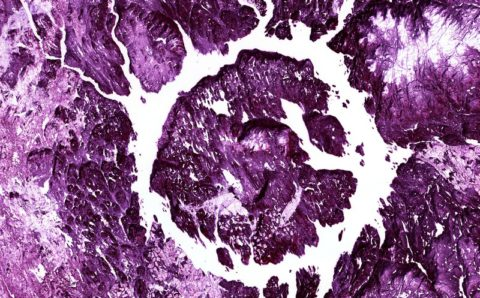Thanks to theidioms.com

## Building a Convolutional Neural Network# Building a Convolutional Neural NetworkBy now we have gained all the basic knowledge required for building a Convolutional Neural Network. Now, it is time to piece it all together.

A Convolutional Neural Network is made up of three main layers:

• The Convolutional Layers
• The Pooling Layers
• The Fully Connected Layers

The following architecture shows how input is passed into the three layers of the Convolutional Neural Network:

## Convolutional Layers in a CNN

The Convolutional Layer is the first layer in a CNN. This layer takes in an input image and performs a series of convolution operations on the image. In other words, the convolutional layer takes in an image tensor as an input, applies a specific number of convolutional filters (kernels) on the image tensor, adds a bias and applies a non-linear activation function (typically, ReLU) to the output.

Till now, we had only applied a single kernel to an image tensor but in a convolutional layer, multiple convolutional filters are used and each filter has its own set of pixel values. However, the process of applying the convolution operation is the same for each filter irrespective of the number of filters used.

The objective of convolutional layers is to extract patterns and informations from an image. The Convolutional filters/kernels at the starting of the network are responsible for capturing the low-level features such as color, gradient orientation, etc. The convolutional filters/kernels deeper down the network are responsible for capturing the high-level features such as edges in the image.

## Pooling Layers in a CNN

The pooling layer is responsible for performing a series of pooling operations (typically, max-pooling) on an image. It takes in an image tensor as an input and outputs a tensor after applying the specified pooling operation.

The main objectives of a pooling layer in a CNN can be summarized as:

• To reduce the computational cost: A pooling layer reduces the size of image tensor, therefore, reducing the number of parameters and computations required in the network.
• To make the network more generic: Pooling helps the network to be more generic because it effectively combines several pixel values into a single one (max-pooling or average pooling). This decreases the chances of the network being biased towards particular pixels (over-fitting).

## Fully Connected Layers in a CNN

The fully connected layer is responsible for taking in a flattened image vector (1-D image tensor) as an input and finding a probability score for each label in the training dataset. The fully connected layer will not be discussed in detail in this chapter since this comes off from the knowledge of the previous course Dense Neural Network Theoretical Course.

This whole architecture makes up a Convolutional Neural Network. The first two layers (convolution and pooling) are responsible for feature extraction from the images so both are also collectively referred to as Feature Extracting Layers, whereas the last fully connected layer is responsible for classifying the image per the task at hand, so also called the Classification Layer.

In a CNN, the convolutional layer and the padding layer can be repeated multiple times before joining at the end with the fully connected layer. Thus, the combination of the convolutional and pooling layer is looked at as a single hidden layer and there can be multiple hidden layers in a CNN.

Are you interested in working with data? Get a free trial month of LinkedIn Learning and learn Data Science and Machine Learning courses using Python, R, and SQL from top instructors.

Enroll in any one of the courses from the following fields today on LinkedIn Learning and start your free month of learning:

Disclaimer: When you subscribe to LinkedIn Learning, we may earn a small commission as an affiliate. We love the platform so much so that we have been using it ourselves at The Click Reader.